# Mean State

Period Mean (original grids) [Watt m-2]
Model Period Mean (intersection) [Watt m-2]
Model Period Mean (complement) [Watt m-2]
Benchmark Period Mean (intersection) [Watt m-2]
Benchmark Period Mean (complement) [Watt m-2]
Bias [Watt m-2]
RMSE [Watt m-2]
Phase Shift [months]
Bias Score 
RMSE Score 
Seasonal Cycle Score 
Spatial Distribution Score 
Interannual Variability Score 
Overall Score 
Benchmark [-] 122.
CLM4 [-] 121. 121. 120. 164. 1.02 17.5 0.914 0.685 0.438 0.880 0.976 0.699 0.686
CLM4.5 [-] 117. 117. 120. 164. -2.83 18.4 0.892 0.665 0.435 0.884 0.934 0.699 0.675
CLM5 [-] 116. 116. 120. 164. -3.73 17.7 0.987 0.682 0.444 0.868 0.893 0.670 0.667
Period Mean (original grids) [Watt m-2]
Model Period Mean (intersection) [Watt m-2]
Model Period Mean (complement) [Watt m-2]
Benchmark Period Mean (intersection) [Watt m-2]
Benchmark Period Mean (complement) [Watt m-2]
Bias [Watt m-2]
RMSE [Watt m-2]
Phase Shift [months]
Bias Score 
RMSE Score 
Seasonal Cycle Score 
Spatial Distribution Score 
Interannual Variability Score 
Overall Score 
Benchmark [-] 102.
CLM4 [-] 103. 102. 101. 165. 1.84 19.4 0.981 0.745 0.497 0.864 0.980 0.708 0.715
CLM4.5 [-] 98.3 97.8 101. 165. -2.66 20.4 1.18 0.655 0.505 0.827 0.860 0.702 0.676
CLM5 [-] 97.7 97.2 101. 165. -3.30 19.8 1.28 0.668 0.513 0.808 0.890 0.651 0.674
Period Mean (original grids) [Watt m-2]
Model Period Mean (intersection) [Watt m-2]
Model Period Mean (complement) [Watt m-2]
Benchmark Period Mean (intersection) [Watt m-2]
Benchmark Period Mean (complement) [Watt m-2]
Bias [Watt m-2]
RMSE [Watt m-2]
Phase Shift [months]
Bias Score 
RMSE Score 
Seasonal Cycle Score 
Spatial Distribution Score 
Interannual Variability Score 
Overall Score 
Benchmark [-] 112.
CLM4 [-] 109. 109. 110. 159. 0.387 20.4 0.939 0.745 0.567 0.867 0.923 0.655 0.721
CLM4.5 [-] 104. 104. 110. 159. -4.74 21.3 0.911 0.680 0.565 0.873 0.851 0.663 0.700
CLM5 [-] 105. 105. 110. 159. -3.73 20.8 1.22 0.712 0.566 0.815 0.873 0.615 0.691
Period Mean (original grids) [Watt m-2]
Model Period Mean (intersection) [Watt m-2]
Model Period Mean (complement) [Watt m-2]
Benchmark Period Mean (intersection) [Watt m-2]
Benchmark Period Mean (complement) [Watt m-2]
Bias [Watt m-2]
RMSE [Watt m-2]
Phase Shift [months]
Bias Score 
RMSE Score 
Seasonal Cycle Score 
Spatial Distribution Score 
Interannual Variability Score 
Overall Score 
Benchmark [-] 43.3
CLM4 [-] 28.6 28.8 43.2 44.3 -15.2 27.1 0.307 0.761 0.665 0.980 0.886 0.768 0.787
CLM4.5 [-] 33.7 33.7 43.2 44.3 -10.9 23.1 0.171 0.812 0.698 0.989 0.792 0.733 0.787
CLM5 [-] 33.3 33.3 43.2 44.3 -11.6 23.4 0.172 0.812 0.698 0.989 0.777 0.762 0.789
Period Mean (original grids) [Watt m-2]
Model Period Mean (intersection) [Watt m-2]
Model Period Mean (complement) [Watt m-2]
Benchmark Period Mean (intersection) [Watt m-2]
Benchmark Period Mean (complement) [Watt m-2]
Bias [Watt m-2]
RMSE [Watt m-2]
Phase Shift [months]
Bias Score 
RMSE Score 
Seasonal Cycle Score 
Spatial Distribution Score 
Interannual Variability Score 
Overall Score 
Benchmark [-] 144.
CLM4 [-] 135. 134. 140. 160. -1.65 19.9 1.09 0.625 0.421 0.841 0.801 0.635 0.624
CLM4.5 [-] 132. 132. 140. 160. -3.26 19.9 1.13 0.633 0.422 0.836 0.872 0.627 0.635
CLM5 [-] 128. 127. 140. 160. -7.88 20.6 0.968 0.595 0.427 0.860 0.898 0.604 0.635
Period Mean (original grids) [Watt m-2]
Model Period Mean (intersection) [Watt m-2]
Model Period Mean (complement) [Watt m-2]
Benchmark Period Mean (intersection) [Watt m-2]
Benchmark Period Mean (complement) [Watt m-2]
Bias [Watt m-2]
RMSE [Watt m-2]
Phase Shift [months]
Bias Score 
RMSE Score 
Seasonal Cycle Score 
Spatial Distribution Score 
Interannual Variability Score 
Overall Score 
Benchmark [-] 62.5
CLM4 [-] 53.1 53.1 62.3 75.7 -8.90 19.5 0.0965 0.849 0.746 0.994 0.843 0.628 0.801
CLM4.5 [-] 50.6 50.6 62.3 75.7 -11.4 21.7 0.0904 0.816 0.730 0.994 0.856 0.638 0.794
CLM5 [-] 48.1 48.1 62.3 75.7 -13.9 23.3 0.107 0.785 0.722 0.993 0.935 0.666 0.804
Period Mean (original grids) [Watt m-2]
Model Period Mean (intersection) [Watt m-2]
Model Period Mean (complement) [Watt m-2]
Benchmark Period Mean (intersection) [Watt m-2]
Benchmark Period Mean (complement) [Watt m-2]
Bias [Watt m-2]
RMSE [Watt m-2]
Phase Shift [months]
Bias Score 
RMSE Score 
Seasonal Cycle Score 
Spatial Distribution Score 
Interannual Variability Score 
Overall Score 
Benchmark [-] 72.7
CLM4 [-] 65.4 65.4 72.1 94.9 -5.48 20.7 0.403 0.798 0.663 0.969 0.970 0.624 0.781
CLM4.5 [-] 64.1 64.0 72.1 94.9 -6.72 21.3 0.404 0.789 0.658 0.968 0.978 0.623 0.779
CLM5 [-] 62.7 62.6 72.1 94.9 -8.19 21.7 0.393 0.791 0.649 0.970 0.991 0.633 0.780
Period Mean (original grids) [Watt m-2]
Model Period Mean (intersection) [Watt m-2]
Model Period Mean (complement) [Watt m-2]
Benchmark Period Mean (intersection) [Watt m-2]
Benchmark Period Mean (complement) [Watt m-2]
Bias [Watt m-2]
RMSE [Watt m-2]
Phase Shift [months]
Bias Score 
RMSE Score 
Seasonal Cycle Score 
Spatial Distribution Score 
Interannual Variability Score 
Overall Score 
Benchmark [-] 109.
CLM4 [-] 99.8 99.4 105. 157. -1.87 23.2 0.448 0.720 0.528 0.957 0.981 0.619 0.722
CLM4.5 [-] 95.6 95.3 105. 157. -5.50 23.3 0.470 0.711 0.531 0.955 0.976 0.604 0.718
CLM5 [-] 94.4 94.1 105. 157. -6.92 23.7 0.544 0.716 0.528 0.952 0.958 0.560 0.707
Period Mean (original grids) [Watt m-2]
Model Period Mean (intersection) [Watt m-2]
Model Period Mean (complement) [Watt m-2]
Benchmark Period Mean (intersection) [Watt m-2]
Benchmark Period Mean (complement) [Watt m-2]
Bias [Watt m-2]
RMSE [Watt m-2]
Phase Shift [months]
Bias Score 
RMSE Score 
Seasonal Cycle Score 
Spatial Distribution Score 
Interannual Variability Score 
Overall Score 
Benchmark [-] 41.8
CLM4 [-] 21.4 21.7 41.9 41.5 -20.7 27.1 0.255 0.678 0.675 0.983 0.954 0.723 0.782
CLM4.5 [-] 24.5 24.9 41.9 41.5 -17.9 24.3 0.292 0.719 0.690 0.981 0.930 0.720 0.788
CLM5 [-] 25.1 25.4 41.9 41.5 -17.5 23.3 0.253 0.725 0.696 0.983 0.940 0.755 0.799
Period Mean (original grids) [Watt m-2]
Model Period Mean (intersection) [Watt m-2]
Model Period Mean (complement) [Watt m-2]
Benchmark Period Mean (intersection) [Watt m-2]
Benchmark Period Mean (complement) [Watt m-2]
Bias [Watt m-2]
RMSE [Watt m-2]
Phase Shift [months]
Bias Score 
RMSE Score 
Seasonal Cycle Score 
Spatial Distribution Score 
Interannual Variability Score 
Overall Score 
Benchmark [-] 96.5
CLM4 [-] 93.7 93.7 93.2 138. 2.21 19.9 0.329 0.781 0.671 0.975 0.859 0.642 0.767
CLM4.5 [-] 93.0 93.0 93.2 138. 1.62 19.9 0.339 0.779 0.669 0.974 0.843 0.652 0.765
CLM5 [-] 92.5 92.4 93.2 138. 1.15 20.2 0.330 0.778 0.666 0.974 0.866 0.637 0.764
Period Mean (original grids) [Watt m-2]
Model Period Mean (intersection) [Watt m-2]
Model Period Mean (complement) [Watt m-2]
Benchmark Period Mean (intersection) [Watt m-2]
Benchmark Period Mean (complement) [Watt m-2]
Bias [Watt m-2]
RMSE [Watt m-2]
Phase Shift [months]
Bias Score 
RMSE Score 
Seasonal Cycle Score 
Spatial Distribution Score 
Interannual Variability Score 
Overall Score 
Benchmark [-] 64.4
CLM4 [-] 56.1 56.2 61.9 90.5 -3.80 19.2 0.270 0.857 0.744 0.982 0.955 0.651 0.822
CLM4.5 [-] 57.7 57.7 61.9 90.5 -2.31 18.2 0.284 0.873 0.748 0.981 0.984 0.652 0.831
CLM5 [-] 54.4 54.4 61.9 90.5 -5.49 19.0 0.289 0.858 0.742 0.981 0.985 0.640 0.825
Period Mean (original grids) [Watt m-2]
Model Period Mean (intersection) [Watt m-2]
Model Period Mean (complement) [Watt m-2]
Benchmark Period Mean (intersection) [Watt m-2]
Benchmark Period Mean (complement) [Watt m-2]
Bias [Watt m-2]
RMSE [Watt m-2]
Phase Shift [months]
Bias Score 
RMSE Score 
Seasonal Cycle Score 
Spatial Distribution Score 
Interannual Variability Score 
Overall Score 
Benchmark [-] 115.
CLM4 [-] 68.2 68.2 77.4 133. -7.29 22.9 0.534 0.708 0.598 0.938 0.928 0.629 0.733
CLM4.5 [-] 67.2 67.2 77.4 133. -8.11 22.8 0.548 0.699 0.599 0.936 0.942 0.627 0.734
CLM5 [-] 65.7 65.7 77.4 133. -10.1 23.7 0.563 0.682 0.597 0.933 0.922 0.623 0.726
Period Mean (original grids) [Watt m-2]
Model Period Mean (intersection) [Watt m-2]
Model Period Mean (complement) [Watt m-2]
Benchmark Period Mean (intersection) [Watt m-2]
Benchmark Period Mean (complement) [Watt m-2]
Bias [Watt m-2]
RMSE [Watt m-2]
Phase Shift [months]
Bias Score 
RMSE Score 
Seasonal Cycle Score 
Spatial Distribution Score 
Interannual Variability Score 
Overall Score 
Benchmark [-] 54.9
CLM4 [-] 54.1 54.2 51.3 143. 4.96 25.9 0.956 0.664 0.522 0.880 0.832 0.541 0.660
CLM4.5 [-] 54.2 54.2 51.3 143. 5.12 25.9 0.973 0.665 0.521 0.878 0.844 0.545 0.662
CLM5 [-] 54.2 54.2 51.3 143. 5.15 26.1 0.880 0.664 0.516 0.890 0.852 0.552 0.665
Period Mean (original grids) [Watt m-2]
Model Period Mean (intersection) [Watt m-2]
Model Period Mean (complement) [Watt m-2]
Benchmark Period Mean (intersection) [Watt m-2]
Benchmark Period Mean (complement) [Watt m-2]
Bias [Watt m-2]
RMSE [Watt m-2]
Phase Shift [months]
Bias Score 
RMSE Score 
Seasonal Cycle Score 
Spatial Distribution Score 
Interannual Variability Score 
Overall Score 
Benchmark [-] 44.9
CLM4 [-] 27.6 27.5 44.8 45.6 -18.7 25.4 0.128 0.716 0.709 0.992 0.934 0.760 0.803
CLM4.5 [-] 31.0 30.9 44.8 45.6 -15.8 22.6 0.129 0.754 0.727 0.991 0.920 0.746 0.811
CLM5 [-] 28.4 28.3 44.8 45.6 -18.6 24.6 0.122 0.720 0.720 0.992 0.875 0.780 0.801
Period Mean (original grids) [Watt m-2]
Model Period Mean (intersection) [Watt m-2]
Model Period Mean (complement) [Watt m-2]
Benchmark Period Mean (intersection) [Watt m-2]
Benchmark Period Mean (complement) [Watt m-2]
Bias [Watt m-2]
RMSE [Watt m-2]
Phase Shift [months]
Bias Score 
RMSE Score 
Seasonal Cycle Score 
Spatial Distribution Score 
Interannual Variability Score 
Overall Score 
Benchmark [-] 134.
CLM4 [-] 108. 109. 127. 169. -13.1 24.2 0.706 0.641 0.601 0.915 0.949 0.659 0.728
CLM4.5 [-] 108. 109. 127. 169. -12.9 24.1 0.704 0.643 0.598 0.916 0.964 0.673 0.732
CLM5 [-] 107. 108. 127. 169. -14.0 24.1 0.799 0.639 0.605 0.898 0.949 0.624 0.720
Period Mean (original grids) [Watt m-2]
Model Period Mean (intersection) [Watt m-2]
Model Period Mean (complement) [Watt m-2]
Benchmark Period Mean (intersection) [Watt m-2]
Benchmark Period Mean (complement) [Watt m-2]
Bias [Watt m-2]
RMSE [Watt m-2]
Phase Shift [months]
Bias Score 
RMSE Score 
Seasonal Cycle Score 
Spatial Distribution Score 
Interannual Variability Score 
Overall Score 
Benchmark [-] 99.6
CLM4 [-] 89.8 90.0 97.8 136. -6.24 17.7 0.276 0.875 0.724 0.978 0.986 0.654 0.824
CLM4.5 [-] 87.1 87.2 97.8 136. -8.79 18.8 0.305 0.833 0.723 0.976 0.986 0.654 0.816
CLM5 [-] 82.0 82.1 97.8 136. -14.0 21.5 0.352 0.754 0.723 0.974 0.998 0.625 0.800
Period Mean (original grids) [Watt m-2]
Model Period Mean (intersection) [Watt m-2]
Model Period Mean (complement) [Watt m-2]
Benchmark Period Mean (intersection) [Watt m-2]
Benchmark Period Mean (complement) [Watt m-2]
Bias [Watt m-2]
RMSE [Watt m-2]
Phase Shift [months]
Bias Score 
RMSE Score 
Seasonal Cycle Score 
Spatial Distribution Score 
Interannual Variability Score 
Overall Score 
Benchmark [-] 72.2
CLM4 [-] 61.8 61.9 72.0 77.2 -10.0 18.3 0.102 0.838 0.771 0.993 0.969 0.646 0.831
CLM4.5 [-] 62.4 62.5 72.0 77.2 -9.43 18.0 0.108 0.845 0.771 0.993 0.992 0.640 0.835
CLM5 [-] 58.6 58.7 72.0 77.2 -13.2 20.6 0.102 0.792 0.759 0.993 0.996 0.667 0.828
Period Mean (original grids) [Watt m-2]
Model Period Mean (intersection) [Watt m-2]
Model Period Mean (complement) [Watt m-2]
Benchmark Period Mean (intersection) [Watt m-2]
Benchmark Period Mean (complement) [Watt m-2]
Bias [Watt m-2]
RMSE [Watt m-2]
Phase Shift [months]
Bias Score 
RMSE Score 
Seasonal Cycle Score 
Spatial Distribution Score 
Interannual Variability Score 
Overall Score 
Benchmark [-] 81.6
CLM4 [-] 73.7 73.9 81.1 116. -6.54 19.2 0.310 0.806 0.717 0.977 0.989 0.677 0.814
CLM4.5 [-] 72.2 72.5 81.1 116. -7.93 20.1 0.296 0.789 0.713 0.978 0.990 0.677 0.810
CLM5 [-] 72.0 72.2 81.1 116. -8.22 20.4 0.314 0.793 0.706 0.977 0.994 0.660 0.806
Period Mean (original grids) [Watt m-2]
Model Period Mean (intersection) [Watt m-2]
Model Period Mean (complement) [Watt m-2]
Benchmark Period Mean (intersection) [Watt m-2]
Benchmark Period Mean (complement) [Watt m-2]
Bias [Watt m-2]
RMSE [Watt m-2]
Phase Shift [months]
Bias Score 
RMSE Score 
Seasonal Cycle Score 
Spatial Distribution Score 
Interannual Variability Score 
Overall Score 
Benchmark [-] 132.
CLM4 [-] 127. 127. 131. 178. -3.71 19.3 0.888 0.725 0.394 0.872 0.910 0.626 0.653
CLM4.5 [-] 124. 124. 131. 178. -6.16 20.1 0.926 0.660 0.393 0.866 0.956 0.624 0.649
CLM5 [-] 122. 122. 131. 178. -7.96 20.3 0.991 0.616 0.395 0.859 0.949 0.603 0.636
Period Mean (original grids) [Watt m-2]
Model Period Mean (intersection) [Watt m-2]
Model Period Mean (complement) [Watt m-2]
Benchmark Period Mean (intersection) [Watt m-2]
Benchmark Period Mean (complement) [Watt m-2]
Bias [Watt m-2]
RMSE [Watt m-2]
Phase Shift [months]
Bias Score 
RMSE Score 
Seasonal Cycle Score 
Spatial Distribution Score 
Interannual Variability Score 
Overall Score 
Benchmark [-] 117.
CLM4 [-] 114. 114. 117. 145. -2.62 19.8 0.957 0.775 0.554 0.867 0.948 0.660 0.726
CLM4.5 [-] 111. 111. 117. 145. -5.30 20.3 0.967 0.738 0.552 0.866 0.941 0.669 0.720
CLM5 [-] 108. 108. 117. 145. -8.04 20.3 0.874 0.730 0.559 0.885 0.968 0.621 0.720
Period Mean (original grids) [Watt m-2]
Model Period Mean (intersection) [Watt m-2]
Model Period Mean (complement) [Watt m-2]
Benchmark Period Mean (intersection) [Watt m-2]
Benchmark Period Mean (complement) [Watt m-2]
Bias [Watt m-2]
RMSE [Watt m-2]
Phase Shift [months]
Bias Score 
RMSE Score 
Seasonal Cycle Score 
Spatial Distribution Score 
Interannual Variability Score 
Overall Score 
Benchmark [-] 95.7
CLM4 [-] 91.7 91.1 92.5 124. -2.48 25.1 0.409 0.742 0.677 0.961 0.987 0.571 0.769
CLM4.5 [-] 90.4 89.8 92.5 124. -3.56 25.1 0.410 0.739 0.678 0.961 0.988 0.572 0.769
CLM5 [-] 90.8 90.1 92.5 124. -3.40 24.9 0.391 0.740 0.681 0.963 0.988 0.568 0.770

# Temporally integrated period mean

BENCHMARK MEAN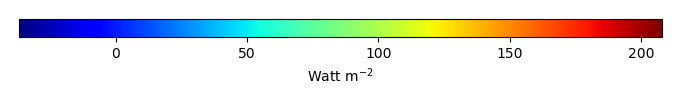MODEL MEANBIAS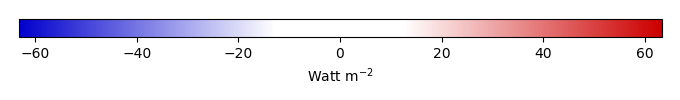BIAS SCORERMSE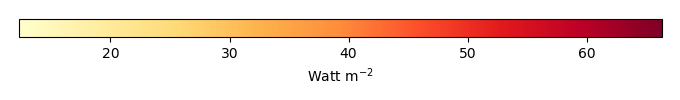RMSE SCOREBENCHMARK INTERANNUAL VARIABILITY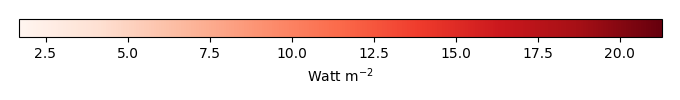MODEL INTERANNUAL VARIABILITYINTERANNUAL VARIABILITY SCOREBENCHMARK MAX MONTHMODEL MAX MONTHDIFFERENCE IN MAX MONTH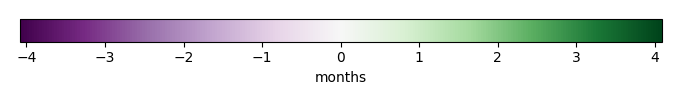SEASONAL CYCLE SCORESPATIAL TAYLOR DIAGRAMMODEL COLORS# Spatially integrated regional mean

MODEL COLORSREGIONAL MEANANNUAL CYCLEMONTHLY ANOMALYANNUAL CYCLE# All Models

BenchmarkCLM4CLM4.5CLM5# Data Information

creation_date: Tue Jul 1 08:16:58 PDT 2014

source_file: This product is generated from monthly 1 degree GEWEX SRB Radiation observations